# 1 函数的定义与调用

## 1.1 函数的定义

• 一个C程序可由一个主函数和若干个其他函数组成
• 程序执行时从main函数开始，根据需要，main函数调用其他函数，其他函数也可以互相调用。
• 同一个函数可以被一个或多个函数调用任意多次。最后由main函数结束程序的运行。
• 不能调用main函数。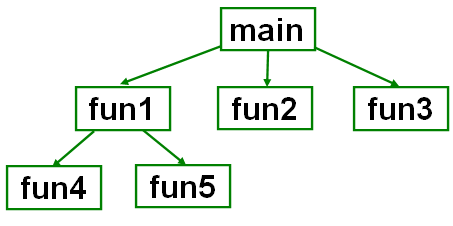例：输入两个整数，输出其中较大的数。
#include<stdio.h>
int max(int x,int y)
{ if(x>y) return x;
else return y;
} // 函数定义。使用return把结果返回主调函数
int main()
{ int a,b,c;
printf("input two numbers:\n");
scanf("%d%d",&a,&b);
c=max(a,b);  //函数调用
printf("max=%d\n",c);
return 0;
}


### 1.1.1 函数的分类

• 从用户的观点分为：
• C编译系统提供的标准库函数
• 用户自定义函数
• 从函数间数据传送的关系分为：
• 有参函数、无参函数
• 有返回值函数、无返回值函数；
• 从函数的调用分为：
• 内部函数和外部函数

### 1.1.2 函数的定义

• 形式：
函数类型 函数名（参数表）——函数头
{ 数据说明
执行语句 } ——函数体
int max(intx,int y)
{ if(x>y) return x;
else return y;
}

• 函数类型
• 指函数值的数据类型
• void -表示函数不返回任何值，称“无类型”或“空类型”；
• 如果省略函数类型，则默认为int型。如：int max（int x, int y）
• 函数名
• 可以是任何有效的标识符；
• 在一个程序中除了主函数外其余函数的名字可以任意取，但应有意义。
• “（）”函数表示
• int max( int x, int y)
• 参数表
• 定义函数时参数表中的参数成为形式参数，用逗号分隔。如，int max（ int x, int y）
• 形式参数可以是变量名、数组名，不允许是常量、表达式或数组元素。如：float fact（n,x[i]）
• 形参省略时称无参函数，但此时函数名后的圆括号不能省。dump( ) { }

• 把同一种类型的参数声明写成：float max(float x,y) 正确的是：float max(float x, float y)
• 在定义函数时，在右圆括号后使用分号。 float max(float x, float y)；
• 函数体
• 用来完成具体任务的一个程序段。
• 结构：数据说明+执行语句
int max(int x,int y)
{ int z;  //本函数体内所用变量的说明
z=x>y?x:y;
return(z);
}

• 注意： 在函数体内不能定义函数，即函数定义不允许嵌套。

## 1.2 函数的返回值与函数类型

• 当被调用函数完成一定的功能后，可将处理的结果返回到调用函数，这种数据传送称为函数的返回值。如果函数有返回值，在函数体内应包含return语句。
• 格式：return(表达式);return 表达式;
• 作用：将表达式的值返回给调用函数；结束被调用函数的执行，并把程序的控制返回到调用它的函数。
int max(int x, int y)
{ int z;
z=x>y?x:y;
return z;  // 返回结果
}

- 当没有返回值时，可以写好才能：
return；}  // 不返回结果

• 注意
• 函数的返回值的类型应与函数的类型一致。如不一致，以函数的类型为准，对返回值进行类型转换，然后传送给调用函数。
int f()
{ return 3.5; }
int main()
{ int a=f(); // a被初始化为3}
• 一个函数可以有多个return语句，但只可能执行其中一个。
int max(int x,int y)
{ if(x>y) return x;
else return y; }

## 1.3 对被调用函数的说明和函数原型

### 函数声明

• 被调用的函数必须存在；
• 标准库函数在调用前，应使用#include;
• 如在使用输入输出函数时，应在头文件开头用#include<stdio.h>
• 使用字符串处理函数，应该用#include<string.h>
• 使用数学库中的函数，应该用#include<math.h>。所有的数学函数返回double型。
• 用户自定义函数在调用前，必须对该函数进行声明；
• 作用：将函数名、函数类型及形参个数、顺序、类型告诉编译器。编译器通过函数声明测试函数调用是否正确。
• 格式：函数类型 函数名(数据类型 形参1, ···);
#include<stdio.h>
float max(float x, float y);
int main()
{ float a,b,c;
scanf("%f%f",&a,&b);
c=max(a,b);
printf("max is %f",c);
return 0;
}

• 函数声明要与函数的定义匹配
• 如果函数的定义在函数调用的后面就需要函数声明；
• 如果被调用函数的定义出现在调用函数之前，可省略函数声明。
#include<stdio.h>
int max(int x,int y)
{ if(x>y) return x; else return y; }
int main()
{ int a,b,c;
scanf("%d%d",&a,&b);
c=max(a,b);
printf("max=%d\n",c);
return 0;
}


## 1.4 函数的调用

C++函数定义和调用过程（超详细）

• 函数的使用是通过函数调用实现的。所谓函数调用就是调用函数向被调用函数传送数据并将控制权交给被调用函数，当被调用函数执行完后，将结果回传给调用函数并交回控制权。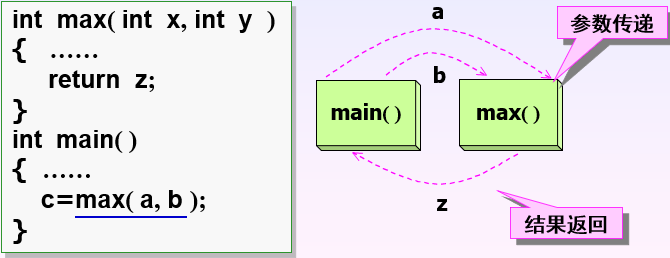• 一般形式调用函数名([实参表])
• 实际参数简称“实参”，是一个具有确定值的表达式。函数在调用时，将实参的值赋给对应的形参。
• 实参表中的实参个数、顺序及类型应与被调用函数中的形参一致。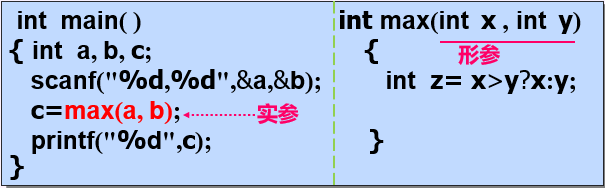• 说明
• 实参可以是常量、变量、表达式、数组元素和数组名；
• 当实参表列中有多个实参时，对实参的求值顺序并不确定，与所用系统有关
• 调用方式
• 函数表达式。c=2*max(a,b);
• 作为语句用（函数语句）。printf("****"); fun();
• 作为函数参数。m=max(a,max(b,c)); printf("%f\n",max(a,b));
#include<stdio.h>
func(int a, int b)
{ int c;
c=a+b;  // 为什么返回值中a=13 b=8？
return c;
}
int main()
{ int x=6,y=7,z=8,r;
r=func((x--,y++,x+y ), z--);
printf("r=%d\n",r); return 0;
}



## 1.5 函数的形参与实参

• 在调用函数时，大多数情况下，调用函数与被调用函数之间有数据传递关系，即实参与形参的结合。
• 正确地进行结合是函数调用的关键。结合时应注意：实参与形参的个数相等，顺序一致，类型应相同

### 形参与实参间的数据传递。

• 实参与形参结合的原则是：
• 当实参为常量、变量、表达式或数组元素时，对应的形参只能是变量名
• 当实参为数组名时，所对应的形参必须是同类型的数组名指针变量
• 形参是变量名时的结合。
• 实参和形参之间的数据传递采用单方向的值传递。只能把实参值传给形参，而不能由形参传回来给实参。在内存中实参单元和形参单元是不同的储存单元。因此，形参的值如发生改变，不会影响到调用函数中的实参的值。
• 特点：单向传递，传递实参的值。实参与形参占用不同的储存单元。
例：实参对形参的数据传递。
#include<stdio.h>
int main()
{ void s(int n);  // 说明函数
int n=100;  // 定义实参n，并初始化
s(n);  // 调用函数
printf("n_s=%d\n",n);  // 输出调用后实参的值，便于进行比较
return 0;
}
void s(int n)
{ int i;
printf("n_x=%d\n",n);  // 输出改变前形参的值
for(i=n-1;i>=1;i--)  // 改变形参的值
n=n+i;
printf("n_x=%d\n",n);  // 输出改变后形参的值
}


# 2 函数的嵌套调用与递归调用

### 2.1 函数的嵌套调用

• 指在执行被调用函数时，被调用函数又调用了其他函数。这与其他语言的子程序嵌套调用的情形是类似的，其关系可表示如下图。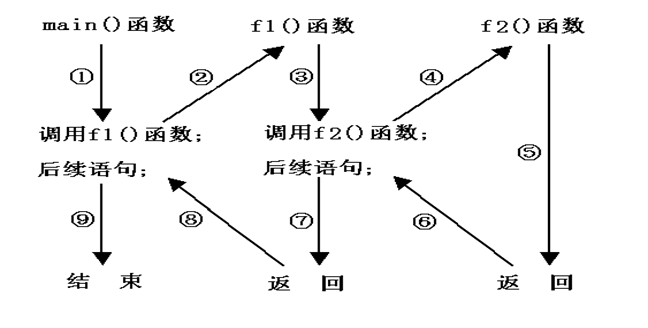• 在c语言中，各函数均处于平等关系，任何一个函数都可以调用和别调用，但main例外。
• 计算：s=1k+2k+3k+…+Nk
#include<stdio.h>
#define K 4
#define N 5
long f1(int n,int k) // 计算n的k次方
{ long power=n;
int i;
for(i=1;i<k;i++)
power*=n;
return power;
}
long f2(int n,int k)  // 计算1到n的k次方之累加和
{ long sum=0;
int i;
for(i=1li<=n;i++)
sum+=f1(i,k);
return sum;
}
main()
{ printf("Sum of %d powers of integers from 1 to %d=", K,N);
printf("%d\n",f2(N,K));
}


### 2.2 函数的递归调用

• 指在一个函数调用过程中有直接或间接调用自己，这样的函数成为递归函数。有直接递归调用和间接递归调用。
• 递归的目的是简化程序，使程序易读
• 递归函数只知道如何去解决最简单的实例（基本实例）
• 简单的返回一个值；
• 把复杂的问题分成两部分：
• 函数知道如何去做的部分；
• 函数不知道如何去做的部分；
• 而这一部分与原问题相似，且更简单；
• 函数可以调用自身来解决这个更小的问题（递归调用）
• 最终解决基本实例
• 函数会沿着调用顺序将一系列的结果返回
• 直到把最终结果放回给原始的调用者（可能是main）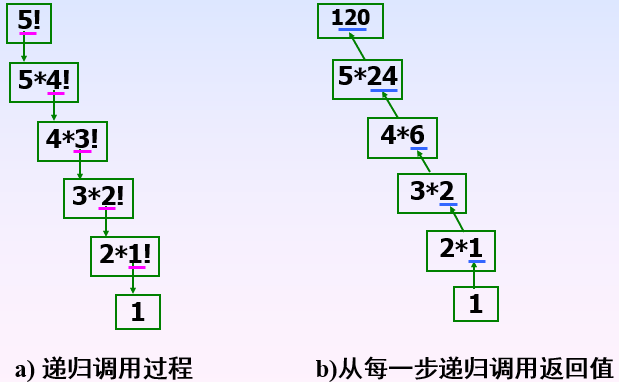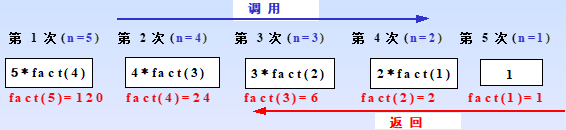#include<stdio.h>
long fact(int)
{ if(n==1) return 1; //递归结束条件
else return(n*fact(n-1));
}
int main()
{ int n;
long f;
printf("n=");
scanf("%d",&n);
f=fact(n);
printf("%d!=%d\n",n,f);
}


# 3 数组作为函数参数

• 数组用作函数参数有两种形式：一种是把数组元素（又称下标变量）作为实参使用；另一种是把数组名作为函数的形参和实参使用。

## 3.1 数组元素作为函数参数

• 数组元素就是下标变量，它与普通变量并无区别。数组元素只能用作函数实参，其用法与普通变量完全相同：在发生函数调用时，把数组元素的值传送给形参，实现单向值传送。
例：写一函数，统计字符串中字母的个数。
#include<stdio.h>
int isalp(char c)
{ if(c>='a'&&c<='z'||c>='A'&&c<='Z')
return(1);
else return(0);
}
int main()
{ int i,num=0;
char str;
printf("Input a string:");
gets(str);
for(i=0;str[i]!='\0';i++)
puts(str);
printf("num=%d\n",num);
return 0;
}

• 说明
• 用数组元素作实参时，只要数组类型和函数的形参类型一致即可，并不要求函数的形参也是下标变量（？？）。换句话说，对数组元素的处理是按普通变量对待的。
• 在普通变量或下标变量作函数参数时，形参变量和实参变量是由编译系统分配的两个不同的内存单元。在函数调用时发生的值传送，是把实参变量的值赋予形参变量。

## 3.2 数组名作为函数的形参和实参

• 应在调用函数和被调函数中分别定义数组，并且实参数组与形参数组的类型必须相同，但大小不要求一致。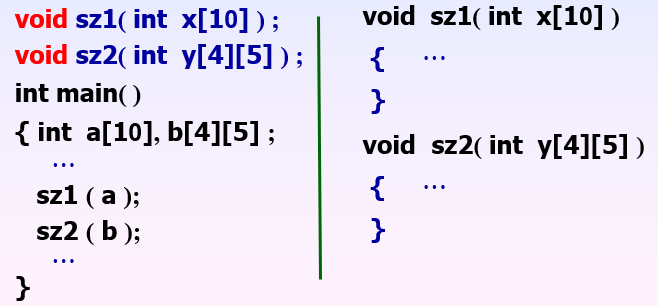• 由于数组名实际上是数组第一个元素的地址，调用函数时，用数组名作实参时，传送给形参是一个地址值，即实参数组的首地址，对应的形参应该是数组名或一个指针变量。
• 实参与形参之间的数据传递是地址传递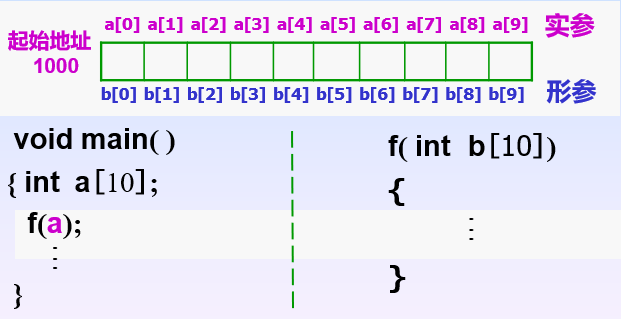• 数组名作为实参时，f 函数中对应的形参可用三种形式进行说明：
• f(int b) ; f(int b[]) ; f(int *b)
例：利用数组进行换数。
#inlcude<stdio.h>
void swap(int x)
{ int t;
t=x; x=x; x=t;
}
void main()
{ int i,a={5,10};
swap(a);
for(i=0;i<2;i++)
printf("a[%d]=%d\n",i,a[i]);
}

• 参数传递的方式：传递实参数组的地址
• 注意：
• 形参数组的长度可以省略；
• 为了在被调用函数中处理数组元素的需要，可另设一个参数传递数组元素的个数。
例：在一维数组score存放10个学生的考试分数，求平均成绩。
#include<stdio.h>
float aver(float a)
{ int i;
float av,sum=a;
for(i=1;i<10;i++)
sum=sum+a[i];
av=sum/10;
return av;
}
int main()
{ float score={100,87,62,93,67,98,95,82,89,90};
float average;
average=aver(score);
printf("平均分：%.1f\n",average);
return 0;
}


## 3.3 用多维数组做函数参数

• 可y用多维数组名作实参和形参。在被调用函数中对形参数组定义时，可以指定每一维的长度，也可省略第一维的长度。如形参数组：int a;int a[];
例：分别计算二维数组a,b的主对角线元素之积p,q，再求p*q。函数名acc。
#include<stdio.h>
int acc(int x[]);  ————→ int acc(int x[],int);
int main()
{ int a,b,i,j,p,q;
for(i=0;i<10;i++)
for(j=0;j<10;j++)
scanf("%d",&a[i][j]);
for(i=0;i<10;i++)
for(j=0;j<10;j++)
scanf("%d",&b[i][j]);
p=acc(a);q=acc(b);  ————→ p=acc(a,3);q=acc(b,10);
printf("result=%d",p*q);
return 0;
}
int acc(int x)  ————→ int acc(int x[],int n)  // 第一括号可以为空，其他下标必须指定
{ int t=x,i;
for(i=1;i<10;i++)  ————→ for(i=1;i<10;n)
t=t*x[i][i];
return t;
}
考虑：若只需要a数组主对角线的前三个元素之积，修改如上

• 小结：

# 4 变量的作用域

• 每个变量都有其作用域（作用范围），所谓变量的作用范围是指变量的“可见性”，即变量在程序中可以存储或引用的范围。
• 根据变量作用域的不同，可分为局部变量和全局变量。

## 4.1 局部变量

• 在一个函数内部定义的变量成为局部变量。
• 作用范围仅限于本函数，即只在本函数范围内有效，其他函数内不能使用。
main(int x,int y) //x,y的作用域从此开始
{ int z;  // z的作用域从此开始
z=x>y?x:y;
return z;
}  //x,y,z的作用域到此结束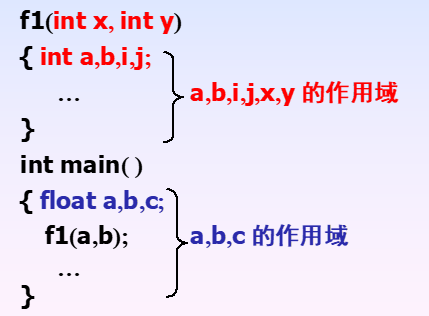• 说明
• main()中定义的变量也只在主函数中有效，而不是在整个文件中有效；主函数不能使用其他函数中定义的变量。
• 不同函数中可使用相同名字的变量，他们代表不同的对象，互不干扰。
• 形参是属于被调用函数的局部变量，实参是属于调用函数的局部变量
• 在函数内部，可在复合语句中定义变量，这个变量只在本复合语句中有效。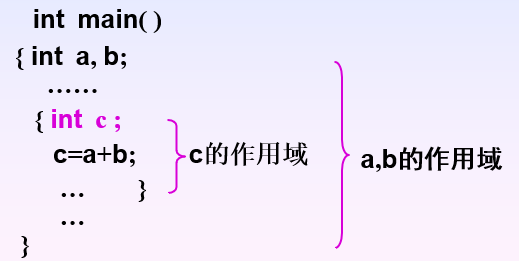## 4.2 全局变量

• 在函数外部定义的变量，也称外部变量。
• 作用范围：是从其定义的地方开始到本源程序文件的结束。
• 全局变量通常在程序顶部定义，其作用范围将覆盖整个源程序文件中各函数。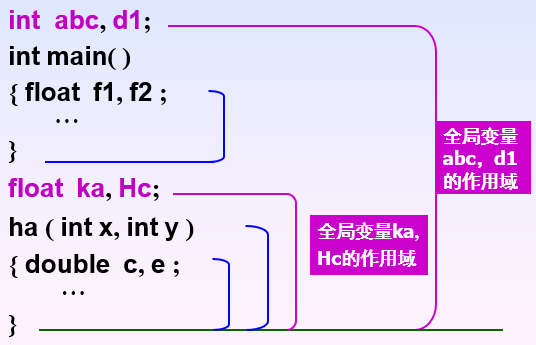• 全局变量的特点：
• 使用全局变量的作用（优点）
• 增加了各函数间数据传送的渠道。函数调用只能返回一个函数值，而利用全局变量可以从函数中得到一个以上的返回值。
• 利用全局变量可以减少函数中实参和形参的个数。
• 缺点：
• 全局变量在程序运行过程中始终占用存储单元，而不是在函数被调用时才临时分配存储单元。
• 使函数的通用性降低。因为函数的运行要依赖于全局变量，这样函数很难进行移植。
• 另外，由于每个函数执行时都有可能改变全局变量的值，程序容易出错。因此，不在必要时不要使用全局变量。
• 在同一个源程序中，若全局变量与局部变量同名，则在局部变量作用范围内，全局变量不起作用，即全局变量被“屏蔽”。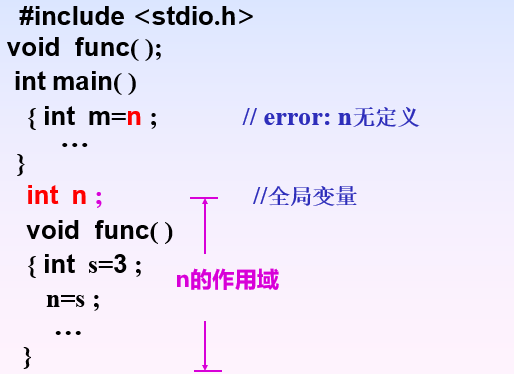• 在统一源文件内，对于使用在前，定义在后的外部变量，可以在使用前用关键字extern加以声明，即可使用。
#include<stdio.h>
void func();
int main()
{ extern int n;  // 外部变量声明，不重新开辟内存哦空间
int m=n;
printf("%d\n",m);
func();
printf("%d\n",n);
return 0;
}
int n;  // 外部变量定义，开辟内存空间
voud func()
{ int s=3;
n=s;
}

0
3


# 5 变量的动态存储与静态存储

• 在c语言中，根据变量的作用域不同，将变量分为局部变量和全局变量。依据变量的生存期不同，则可将变量分为静态存储方式和动态存储方式，具体可分为4种存储类型：自动变量（auto）、寄存器变量（register）、外部变量（extern）、静态变量（static）。自动变量和寄存变量属于动态存储方式，外部变量和静态内部变量属于静态存储方式。
• 全局变量都是静态方式存储，局部变量通常是动态方式存储，但用static声明的局部变量是静态方式存储。

## 5.1 内部变量的存储方式

• 静态存储——静态内部变量
• 定义格式：static 数据类型 内部变量表；
• 存储特点
• 静态内部变量属于静态存储。在程序执行过程中，即使所在函数调用结束也不释放。换句话说，在程序执行期间，静态内部变量始终存在，但其他函数是不能引用它们的。
• 定义但不初始化，则自动赋以“0”（整型和实型）或’\0’（字符型）；但每次调用它们所在的函数时，不再重新赋值，只是保留上次调用结束时的值！
• 何时使用静态内部变量
• 需要保修函数上一次调用结束时的值。
• 变量只被引用而不改变其值。
• 动态存储——自动局部变量（又称自动变量）
• 定义格式：[auto] 数据类型 变量表；
• 存储特点
• 自动变量属于动态存储方式。在函数中定义的自动变量，只在该函数内有效；函数被调用时分配存储空间，调用结束就释放。在复合语句中定义的自动变量，只在该复合语句中有效；退出复合语句后，也不能再使用，否则将引起错误。
• 定义而不初始化，则其值是不确定的。如果初始化，则赋初值操作是在调用时进行的，且每次调用都要重新赋一次初值。
• 由于自动变量的作用域和生存期，都局限于定义它的个体内（函数或复合语句），因此不同的个体中允许使用同名的变量而不会混淆。即使在函数内定义的自动变量，也可与该函数内部的复合语句中定义的自动变量同名。
#include<stdio.h>
void f(int c)
{ int a=0;  // 每次调用时，都会对变量a初始化，不保留上一次的值
static int b=0;  // 只对静态局部变量b初始化一次
a++; b++;
printf("%d:a=%d,b=%d\n",c,a,b);
}
int main()
{ int i;
for(i=1;i<3;i++)
f(i);  // 调用两次函数
return 0；
}

1:a=1,b=1
2:a=1,b=2
3:a=1,b=3

• 静态变量的特点
• 静态局部变量和全局变量一样，只赋初值一次，以后每次函数调用时使用上次函数调用结束时的保留值。
• 静态局部变量定义时如不赋初值，系统会自动对数值型静态局部变量赋初值0；对字符型赋初值空字符。
• 静态局部变量仅能为本函数使用，其他函数不能使用和影响它们。
• 自动变量和静态局部变量区别
• 自动变量的值在函数调用结束后不再保留，下次调用不能使用已有的值。
• 静态局部变量在函数调用结束后，仍然保留上次调用结束的值。
• 寄存器存储——寄存器变量
• 一般情况下，变量的值都是存储在内存中的。为提高执行效率，C语言允许将局部变量的值存放到寄存器中，这种变量就成为寄存器变量。
• 定义格式：register 数据类型 变量表;
• 只有局部变量才能定义成寄存器变量，即全局变量不行。
• 对寄存器变量的实际处理，随系统而异。例如，微机上的MSC和TC将寄存器变量实际当作自动变量处理。
• 允许使用的寄存器数目是有限的，不能定义任意多个寄存器变量。

## 5.2 外部变量的存储方式

• 外部变量属于静态存储方式
• 静态外部变量——只允许被本源文件中的函数引用。定义格式：static 数据类型 外部变量表;
• 非静态外部变量——允许被其他源文件中的函数引用。定义格式：extern 数据类型 外部变量表;
• 定义时缺省static关键字的外部变量，即为非静态外部变量。其他源文件中的函数，引用非静态外部变量时，需要在应用函数所在的源文件中进行格式说明。
• 静态局部变量和静态外部变量同属静态存储方式，但两者区别较大：
• 定义的位置不同。静态局部变量在函数定义，静态外部变量在函数外定义。
• 作用域不同。静态局部变量属于内部变量，其作用域仅限于它的函数；虽然生存期为整个源程序，但其他函数时不能使用它的。静态外部变量在函数外定义，其作用域为定义它的源文件内；生存期为整个源程序，但其它源文件中的函数也是不能使用它。
• 初始化处理不同。静态局部变量，仅在第1次调用它所在的函数时被初始化，当再次调用定义它的函数时，不再初始化，而是保留上1次调用结束时的值。而静态外部变量是在函数外定义的，不存在静态内部变量的“重复”初始化问题，其当前值由最近1次给它赋值的操作决定。

# 6 内部函数与外部函数

• 当一个源程序由多个源文件组成时，C语言根据函数能否被其它源文件中的函数调用，将函数分为内部函数外部函数

## 6.1 内部函数（又称静态函数）

• 如果在一个源文件中定义的函数，只能被本文件中的函数调用，而不能被统一程序其它文件中的函数调用，这种函数成为内部函数。
• 定义一个内部函数，只需在函数类型前加一个“static”关键词即可，如下所示：
static 函数类型 函数名（函数参数表）
{…}
• 关键字"static"，译成中文就是“静态的”，所以内部函数又称静态函数。但此处“static”的含义不是指存储方式，而是指对函数的作用域仅局限于本文件。
• 使用内部函数的好处是：不同的人编写不同的函数时，不同担心自己定义的函数，是否会与其他文件中的函数同名，因为同名也没有关系。

## 6.2 外部函数

• 在定义函数时，如果没有加关键字“static”，或冠以关键字“extern”，表示此函数是外部函数，格式为：
[extern] 函数类型 函数名（函数参数表）
{…}
• 调用外部函数时，需要对其进行声明，格式为：
[extern]函数类型 函数名（参数类型表）[，函数名2（参数类型表2）…]；
例：外部函数应用。
（1）文件mainf.c
main()
{ extern void input(...), process(...), output(...);
input(...);process(...);output(...);
}
（2）文件subf1.c
……
extern void input(……)			/*定义外部函数*/
{……}
（3）文件subf2.c
……
extern void process(……)			/*定义外部函数*/
{……}
（4）文件subf3.c
……
extern void output(……)			/*定义外部函数*/
{……}


# 7 多个源程序文件的编译和连接

04-25146
03-08215805-251万+
09-202535
08-312886
07-1016
05-01107
04-25670
05-141242